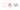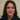# Javascript ES6 maps and how to use them in practice

There has never been a good data structure in Javascript such as ES6 maps for mapping values to values. The key thing about maps are:

• You could use any data type even object type for key value pairs which is not possible if you use objects( Objects use strings, integer and symbols only)
• As the name map suggests you can have a look-up map structure to find values for your unique keys with special iteration

## Define a map

This is how we could create an ES6 map:

`var map = new Map(); //Empty Map`
`var map = new Map([[1,2],[2,3]]); // map = {1=>2, 2=>3}`

Map actually creates an iterable array with elements of key-value pairs.

### Create a map and set the values

Lets create some examples and see some map methods:

Create the map object(Yes map is an object itself):

`var myMap = new Map();`

As mentioned before we could have different type of key-value pairs. We create three different types here:

`var keyString = 'a string',`
`keyObj = {},`
`keyFunc = function() {};`

And we set thier values using the set method:

`myMap.set(keyString, "value associated with 'a string'");`
`myMap.set(keyObj, 'value associated with keyObj');`
`myMap.set(keyFunc, 'value associated with keyFunc');`

WE could check the map size (similar to length) using the size method:

`myMap.size; // 3`

### Get the key values

We could get the values using the get method:

`myMap.get(keyString); // "value associated with 'a string'"`
`myMap.get(keyObj); // "value associated with keyObj"`
`myMap.get(keyFunc); // "value associated with keyFunc"`

`myMap.get('a string'); // "value associated with 'a string'"`
`// because keyString === 'a string'`

In the below examples,we get undefined result because the value specified is not found as keys in the map.

`myMap.get({}); // undefined, because keyObj !== {}`
`myMap.get(function() {}) // undefined, because keyFunc !== function () {}`

Iterate over maps using for.. of

We could iterate through maps using for… of loop in different ways. Check this example:

`var myMap = new Map();`
`myMap.set(0, 'zero');`
`myMap.set(1, 'one');`

As a key-value pair:

``````for (var [key, value] of myMap) {`
`console.log(key + ' = ' + value);`
`}`
`// 0 = zero`
`// 1 = one`

Only keys:

`for (var key of myMap.keys()) {`
`console.log(key);`
`}`
`// 0`
`// 1`

Iterating through values:

`for (var value of myMap.values()) {`
`console.log(value);`
`}`
`// zero`
`// one`

And using entries method to loop through key-value pairs and log them out:

`for (var [key, value] of myMap.entries()) {`
`console.log(key + ' = ' + value);`
`}`
`// 0 = zero`
`// 1 = one`

### Using forEach loop to iterate maps

Like any object maps have built-in forEach method for iteration:

`myMap.forEach(function(value, key) {`
`console.log(key + ' = ' + value);`
`});`
`// Will show 2 logs; first with "0 = zero" and second with "1 = one"`

## Maps and arrays

ES6 maps look like arrays but they are not arrays. However as mentioned about using Array.form around NodeLists in [this post](https://www.nikpro.com.au/javascript-nodelist-and-html-dom-collection-and-how-to-use-them/), we could convert them to each other:

Create an array:

`var kvArray = [['key1', 'value1'], ['key2', 'value2']];`

We use the regular Map constructor to transform a 2D key-value Array into a map:``````

var myMap = new Map(kvArray);`

`myMap.get('key1'); // returns "value1"`

We could also use the Array.from function to transform a map into a 2D key-value Array:

`console.log(Array.from(myMap)); // Will show you exactly the same Array as kvArray`

Also we could use the keys or values iterators and convert them to an array:

`console.log(Array.from(myMap.keys())); // Will show ["key1", "key2"]`

Now lets clone a map just like arrays. The data itself is not cloned:

`var original = new Map([`
`[1, 'one']`
`]);`

`var clone = new Map(original);`

`console.log(clone.get(1)); // one`
`console.log(original === clone); // false. Useful for shallow comparison`

We could merge maps keeping the uniqueness of the keys. This is a good example:

`var first = new Map([`
`[1, 'one'],`
`[2, 'two'],`
`[3, 'three'],`
`]);`

`var second = new Map([`
`[1, 'uno'],`
`[2, 'dos']`
`]);`

We merge two maps. The last repeated key wins which in here the second one is the winner. The ES6 spread operator essentially converts a Map to an Array:

`var merged = new Map([...first, ...second]);`

`console.log(merged.get(1)); // uno`
`console.log(merged.get(2)); // dos`
`console.log(merged.get(3)); // three`

Lets use the same approach to merge those maps with an array and again the last repeated key wins. (the last pair is for the array then):

`var merged = new Map([...first, ...second, [1, 'eins']]);`

`console.log(merged.get(1)); // eins, the array wins here as it comes last in merge`
`console.log(merged.get(2)); // dos`
`console.log(merged.get(3)); // three`

This is another example of using all methods of maps:

`const map = new Map()`

`map.set(5, "Hello")`
`map.set("5", "World")`
`map.set("John", "The revelator")`
`map.size // 3`
`// Map { 5 => 'Hello', '5' => 'World', 'John' => 'The revelator' }`

`map.get(5) // Hello`
`map.has('5') // true`
`map.get('Random') // undefined`
`map.has('John') // true`

`map.delete('5')`
`map.size // 2`
`// Map { 5 => 'Hello', 'John' => 'The revelator' }`

`map.clear()`
`map.size // 0`
`// Map {}`

### Objects in ES6 maps

You could also use objects in maps and it works just perfect:

`const map = new Map()`

Create two objects:

`let obj1 = {}`
`let obj2 = {}`

Assign them to the map:

`map.set(obj1, 12)`
`map.set(obj2, "OBJECT")`
`map.size // 2`

Now the map looks like this:

`console.log(Array.from(map));`

`// Map { {} => 12, {} => 'OBJECT' }`

With these examples we now have abetter idea about ES6 maps.How to create and manipulate them. You can also refer to this article about maps versus objects and this one for details and this one  for a real world example. Thanks for reading.